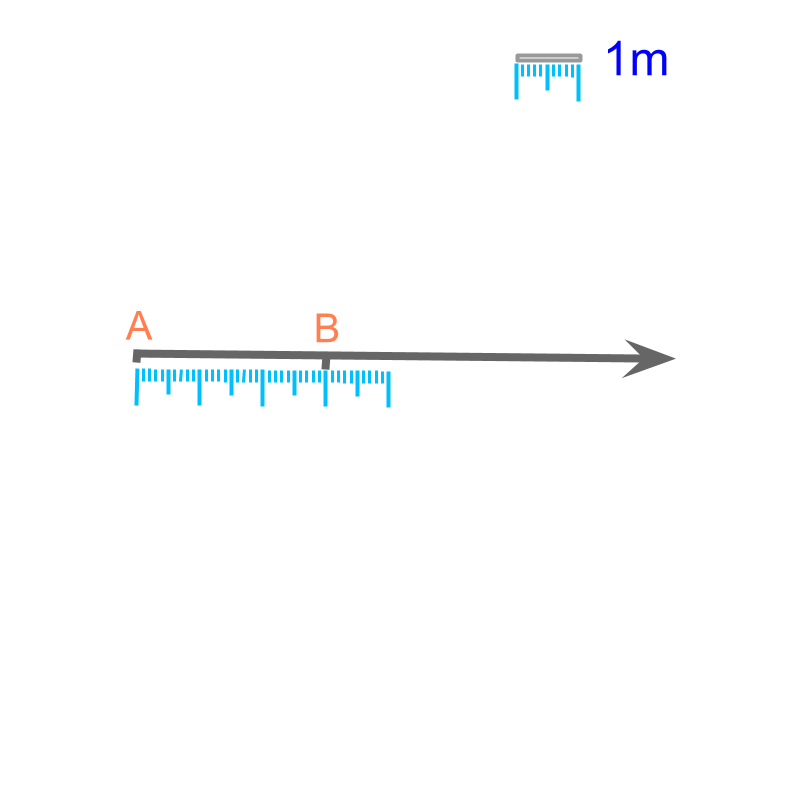maths > mensuration-basics

Introduction to Measuring Length

what you'll learn...

Overview

Length : The distance-span between two points is the length. It is measured in meter or in one of its other forms.Length is specified as a number in reference to the reference-prototype-standard meter (or in one of other derived or similar forms).

distance-span

Length is "the measurement of distance-span between two points, specified in reference to the standard meter"

The word "span" means: full extent or full spread of something.Length of an object is the distance-span of the object from one end to the other end. To specify the distance-span the standard-unit meter is defined. The figure illustrates reference $1m$$1 m$ at the top-right corner and the length of line segment $\overline{AB}$$\overline{A B}$ is measured as $3m$$3 m$ in reference to the standard-unit.

The standard unit of measure for length is "meter"

large or small

The distance between two cities is $200000$$200000$ meter. It is noted that the number is very large in some applications.

To simplify specifying such large values, a larger measure of length is defined such as $1$$1$ kilometer = $1000$$1000$ meter. Using the larger measure, the distance between two cities is given as $200$$200$ kilometer.

The length of a pen is very small to measure in $1$$1$ meter. It is noted that the number is very small in some applications.

To simplify specifying such small values, a smaller measure of length is defined such as $1$$1$ meter = $100$$100$ centimeter. Using the smaller measure, the length of a pen is given as $7$$7$ centimeter.

The basic unit "meter" is converted into smaller or larger units of lengths.

$1000$$1000$ millimeter = $1$$1$ meter

$100$$100$ centimeter = $1$$1$ meter

$10$$10$ decimeter = $1$$1$ meter

$1$$1$ decameter = $10$$10$ meter

$1$$1$ hectometer = $100$$100$ meter

$1$$1$ kilometer = $1000$$1000$ meter Remember the following:
"milli" is from a Latin root word meaning thousand
"centi" is from a Latin root word meaning hundred
"deci" is from a Latin root word meaning tenth
"deca" is from a Greek root word meaning ten
"hecto" is from a Greek root word meaning hundred
"kilo" is from a Greek root word meaning thousand

Among these, millimeter, centimeter, meter, and kilometer are widely used depending on the scale of the length measurement.

what it means

The statement "length of the stick is $3$$3$ meter"
specifies that
"The distance-span of the stick equals $3$$3$ distance-spans given by the standard unit meter".

summary

Length : The distance-span between two points is the length. It is measured in meter or in one of its other forms.Length is specified as a number in reference to the reference-prototype-standard meter (or in one of other derived or similar forms).

Outline

The outline of material to learn "Mensuration basics : Length, Area, & Volume" is as follows.

•  Measuring Basics

→   Introduction to Standards

→   Measuring Length

→   Accurate & Approximate Meaures

→   Measuring Area

→   Measuring Volume

→   Conversion between Units of Measure

•  2D shapes

→   Perimeter of Polygons

→   Area of Square & rectangle

→   Area of Triangle

→   Area of Polygons

→   Perimeter and area of a Circle

→   Perimeter & Area of Quadrilaterals

•  3D shapes

→   Surface Area of Cube, Cuboid, Cylinder

→   Volume of Cube, Cuboid, Cylinder